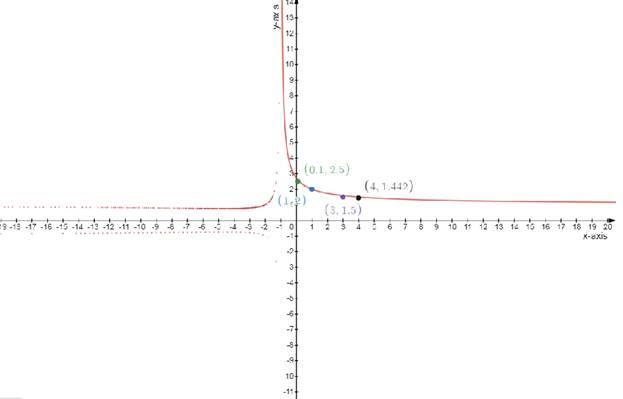# The value of given limit at that every point .### Single Variable Calculus: Concepts...

4th Edition
James Stewart
Publisher: Cengage Learning
ISBN: 9781337687805### Single Variable Calculus: Concepts...

4th Edition
James Stewart
Publisher: Cengage Learning
ISBN: 9781337687805

#### Solutions

Chapter 2.2, Problem 27E

(a)

To determine

## To find:The value of given limit at that every point .

Expert Solution

limx0f(x)=2.71828

### Explanation of Solution

Given:

The function f is

limx0(1+x)1x

Concept used:

Let f(x) be a function defined on an interval that contains x=a , except possibly at x=a

Then

limxaf(x)=L

Calculation:

The existence of the limit

limx0(1+x)1x

Apply by the limit and using the value of the given limit

limx0f(x)=limx0(1+x)1xlimx0f(x)=elimx0f(x)=2.71828

(b)

To determine

Expert Solution

### Explanation of Solution

Given:

The function f is

limx0(1+x)1x

Concept used:

Let f(x) be a function defined on an interval that contains x=a , except possibly at x=a

Then

limxaf(x)=L

Calculation:

The existence of the limit

f(x)=(1+x)1x

Test one point in each of the region formed by the graph

If the point satisfies the function then shade the entire region to denote that every point in the region satisfies the function .

To draw the table

f(x)=(1+x)1x

 x−axis 1 0.1 3 4 y−axis 2 2.5 1.5 1.44

To draw a graph### Have a homework question?

Subscribe to bartleby learn! Ask subject matter experts 30 homework questions each month. Plus, you’ll have access to millions of step-by-step textbook answers!